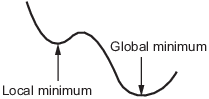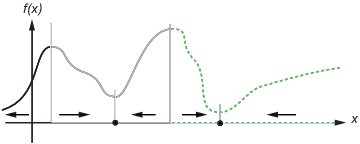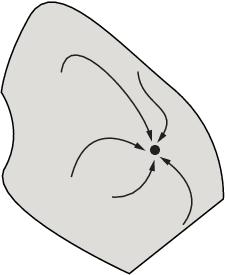What Is Global Optimization?

Local vs. Global Optima

Optimization is the process of finding the point that minimizes a function. More specifically:

• A local minimum of a function is a point where the function value is smaller than or equal to the value at nearby points, but possibly greater than at a distant point.

• A global minimum is a point where the function value is smaller than or equal to the value at all other feasible points.Generally, Optimization Toolbox™ solvers find a local optimum. (This local optimum can be a global optimum.) They find the optimum in the basin of attraction of the starting point. For more information, see Basins of Attraction.

In contrast, Global Optimization Toolbox solvers are designed to search through more than one basin of attraction. They search in various ways:

• GlobalSearch and MultiStart generate a number of starting points. They then use a local solver to find the optima in the basins of attraction of the starting points.

• ga uses a set of starting points (called the population) and iteratively generates better points from the population. As long as the initial population covers several basins, ga can examine several basins.

• particleswarm, like ga, uses a set of starting points. particleswarm can examine several basins at once because of its diverse population.

• simulannealbnd performs a random search. Generally, simulannealbnd accepts a point if it is better than the previous point. simulannealbnd occasionally accepts a worse point, in order to reach a different basin.

• patternsearch looks at a number of neighboring points before accepting one of them. If some neighboring points belong to different basins, patternsearch in essence looks in a number of basins at once.

• surrogateopt begins by quasirandom sampling within bounds, looking for a small objective function value. surrogateopt uses a merit function that, in part, gives preference to points that are far from evaluated points, which is an attempt to reach a global solution. After it cannot improve the current point, surrogateopt resets, causing it to sample widely within bounds again. Resetting is another way surrogateopt searches for a global solution.

Basins of Attraction

If an objective function f(x) is smooth, the vector –∇f(x) points in the direction where f(x) decreases most quickly. The equation of steepest descent, namely

$\frac{d}{dt}x\left(t\right)=-\nabla f\left(x\left(t\right)\right),$

yields a path x(t) that goes to a local minimum as t gets large. Generally, initial values x(0) that are close to each other give steepest descent paths that tend to the same minimum point. The basin of attraction for steepest descent is the set of initial values leading to the same local minimum.

The following figure shows two one-dimensional minima. The figure shows different basins of attraction with different line styles, and it shows directions of steepest descent with arrows. For this and subsequent figures, black dots represent local minima. Every steepest descent path, starting at a point x(0), goes to the black dot in the basin containing x(0).The following figure shows how steepest descent paths can be more complicated in more dimensions.The following figure shows even more complicated paths and basins of attraction.Constraints can break up one basin of attraction into several pieces. For example, consider minimizing y subject to:

• y ≥ |x|

• y ≥ 5 – 4(x–2)2.

The figure shows the two basins of attraction with the final points.The steepest descent paths are straight lines down to the constraint boundaries. From the constraint boundaries, the steepest descent paths travel down along the boundaries. The final point is either (0,0) or (11/4,11/4), depending on whether the initial x-value is above or below 2.

Global Optimization Toolbox DocumentationGet trial now